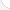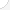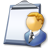Medical scientistsDescription: Medical scientists research human diseases in order to improve human health. Most medical scientists do basic research to learn more about viruses, bacteria, and other infectious diseases. Then this information is used to develop vaccines, medicines, and treatments for many diseases.Source: Bureau of Labor Statistics, U.S. Department of Labor, Occupational Outlook Handbook, 2008-09 Edition at http://www.bls.gov/OCO/Complete Job Profile: http://www.bls.gov/oco/ocos008.htmSalary: \$50,001 or more per yearComments:

There are 49 math topics Medical scientists need to know.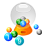Basic Math / Algebra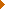FractionsDecimalsRatio and ProportionPercentCustomary MeasurementMetric MeasurementMeasurement ConversionBasic ProbabilityBasic StatisticsStatistical GraphingPowers and RootsOther Number BasesNegative NumbersScientific NotationBasic Problem Solving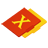First-Year AlgebraUsing FormulasLinear EquationsRational ExpressionsCoordinate Graphing 2DLinear SystemsQuadratic EquationsAlgebraic Representation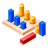GeometryBasic TerminologySimilarityGeometric MeanAreaVolumeTransformationsMake/Use 3D Drawings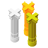Second-Year Algebra / TrigonometryFunctionsVariationLogarithmsSequences and SeriesCoordinate Graphing 3DAdvanced ProbabilityAdvanced StatisticsTrigonometric/Circular FunctionsGraphs of Trigonometric FunctionsPolar Coordinates/GraphsVectors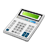Other TopicsCalculus and Higher MathBasic Calculator UseScientific Calculator UseComputer UseGroup Problem SolvingMental MathInductive/Deductive ReasoningMath CommunicationsMathematical Modeling